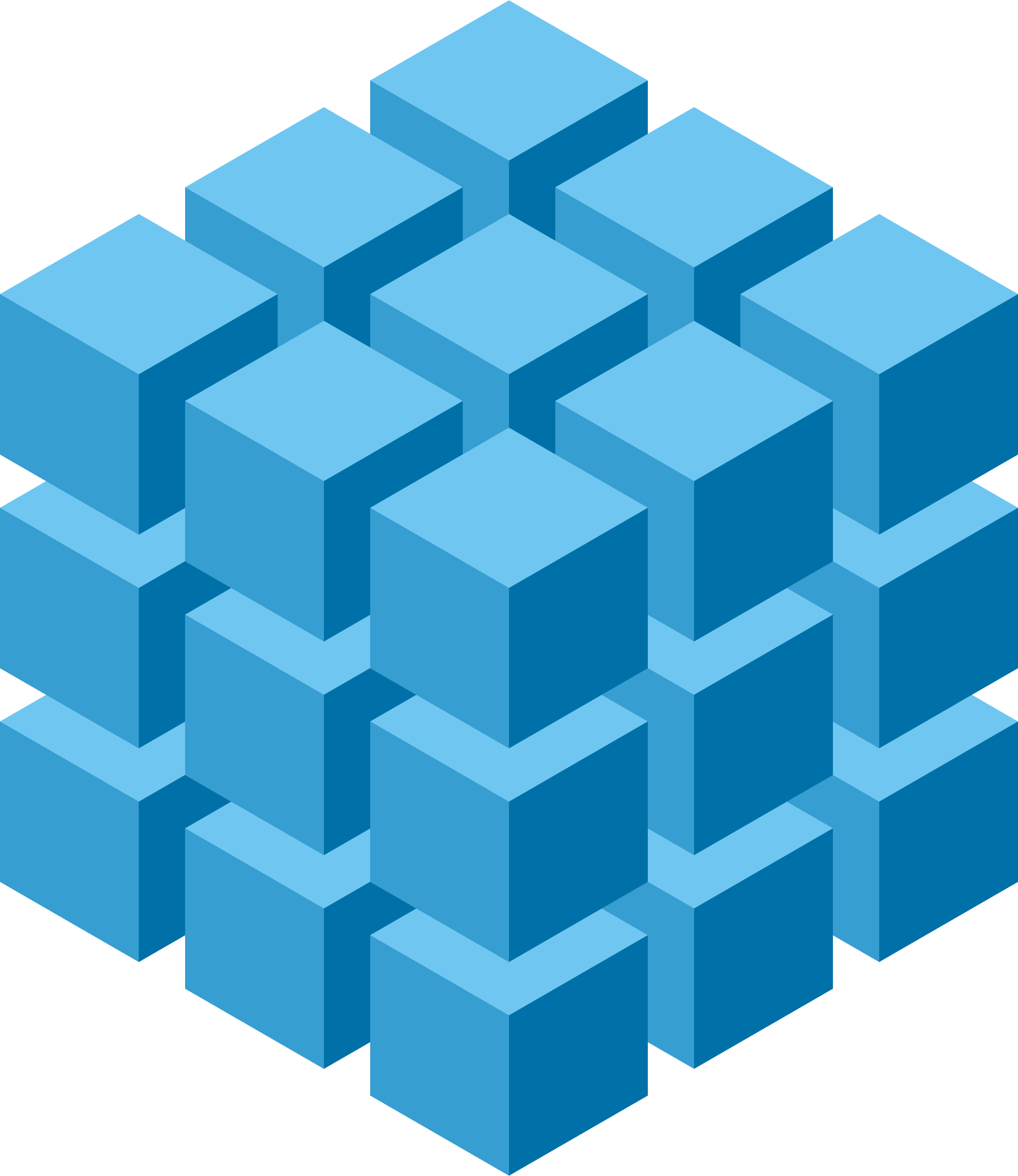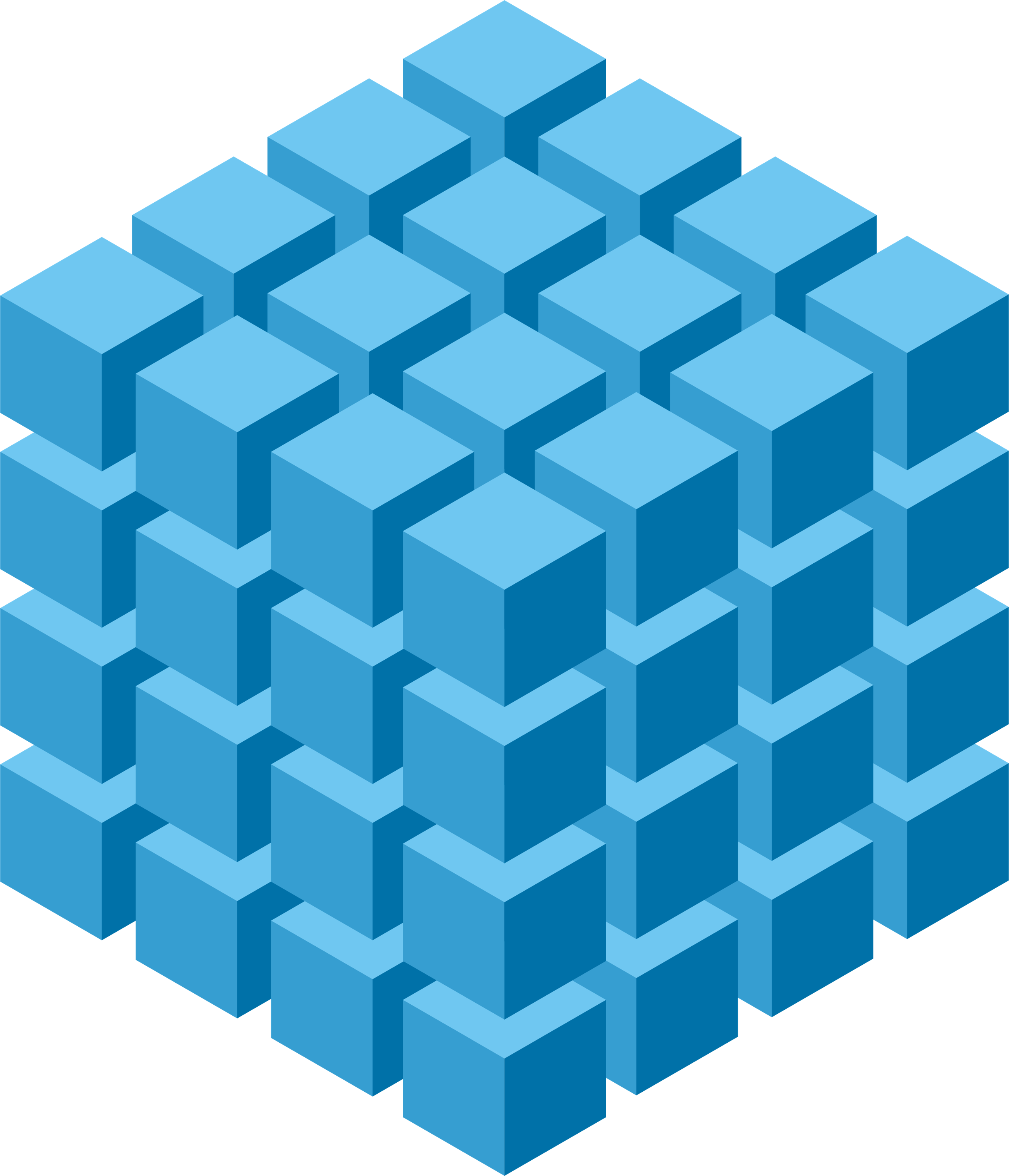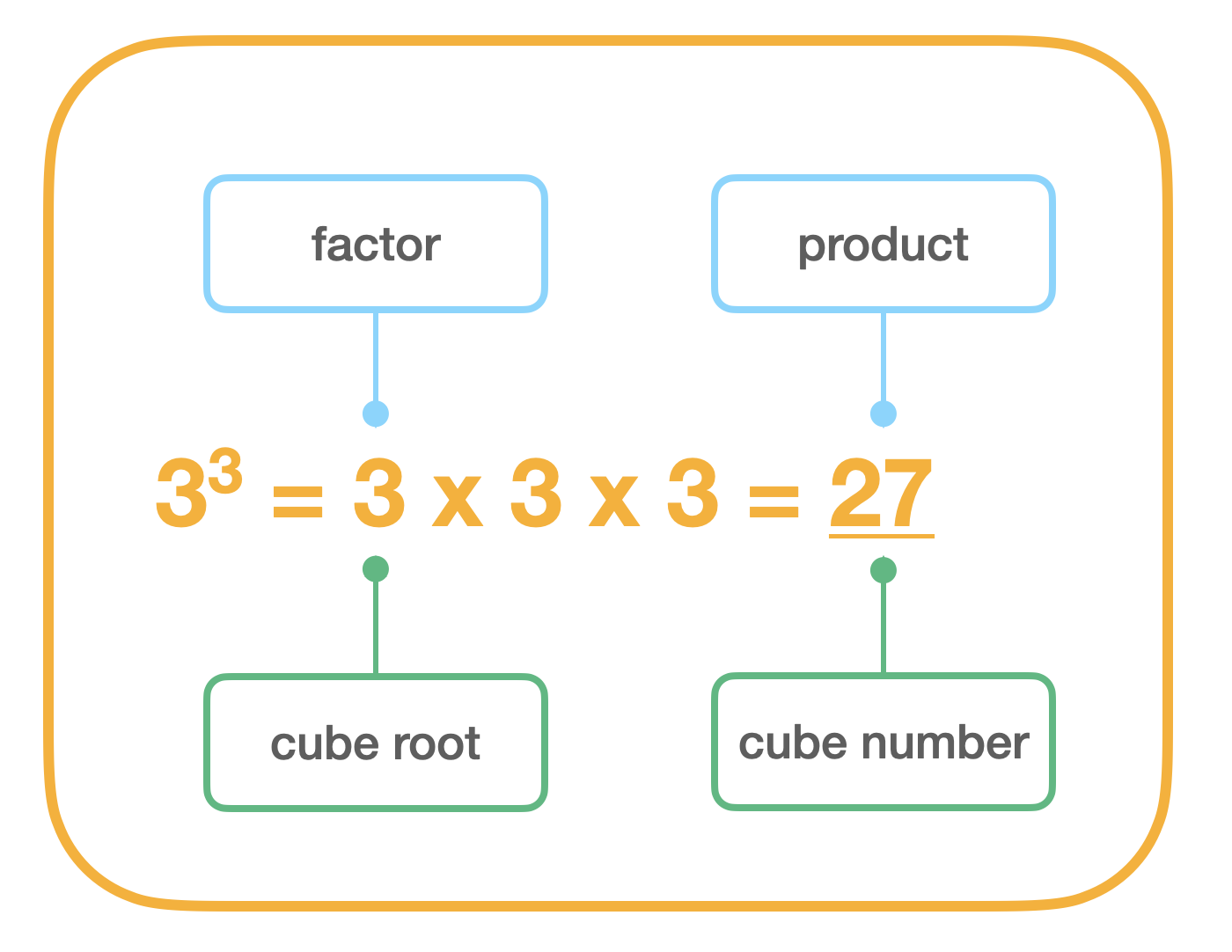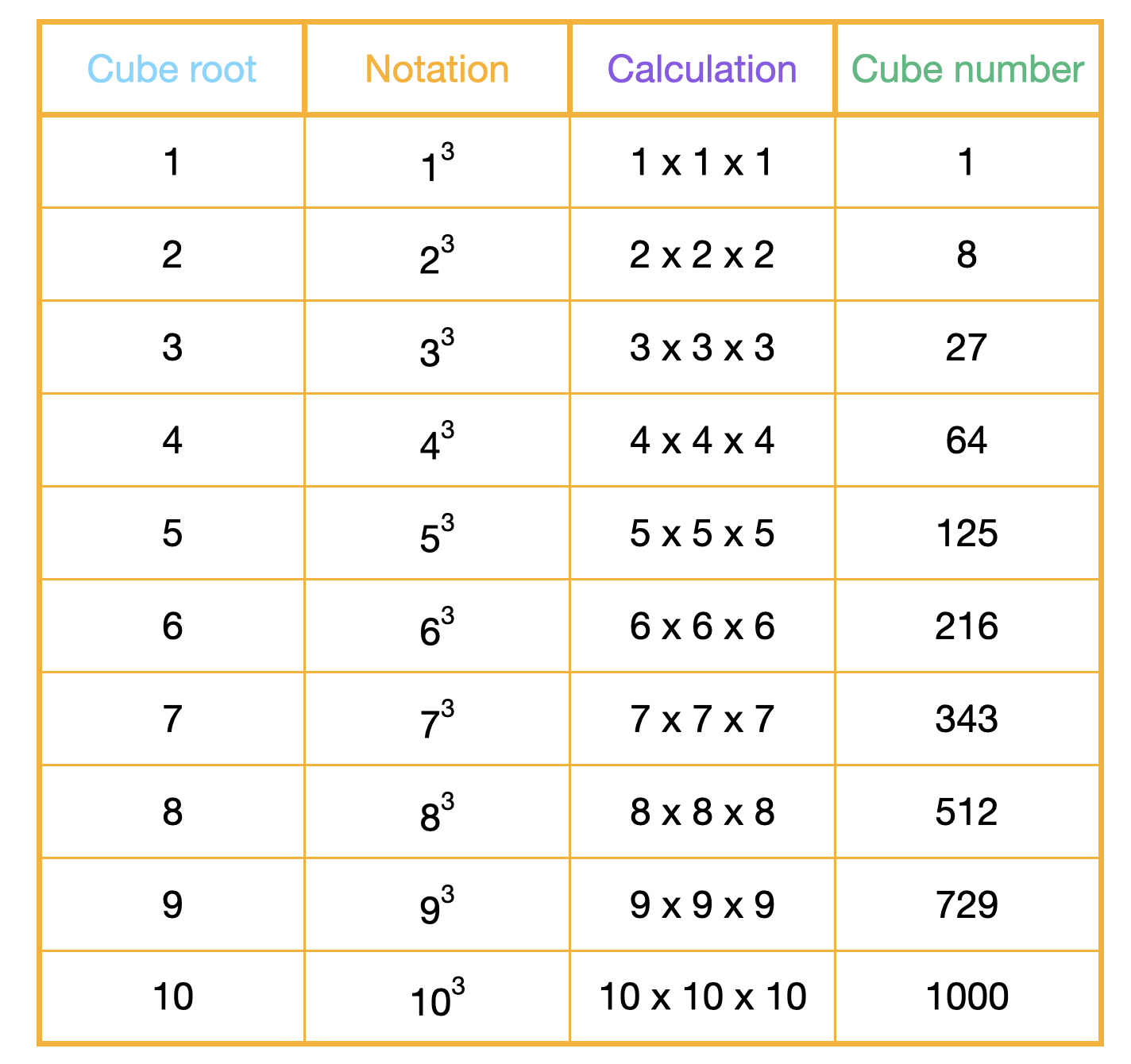# Cube numbers

## What are cube numbers?

A cube number is the product of three identical factors (e.g. 2 x 2 x 2 = 8). So, a cube number is any number which has been multiplied by itself, twice.

Sometimes, the language 'cubed' is used (e.g. '2 cubed' = 2 x 2 x 2 = 8).

## How to calculate cube numbers

Calculating a cube number is simple. To generate a cube number, multiply a number by itself. Then, multiply the product by the number again!23 = 2 x 2 x 2

2 x 2 = 4

4 x 2 = 8

33 = 3 x 3 x 3

3 x 3 = 9

3 x 3 = 27

43 = 4 x 4 x 4

4 x 4 = 16

16 x 4 = 64

## Why are cube numbers called cube numbers?

Cube numbers are called cube numbers (or 'cubed numbers') because they can be visually represented as a three-dimensional cube: a shape with an equal number of units along its length, width, and height.

## Cube number notation

The symbol to demonstrate that a number is cubed is 3 (e.g. 23 =8). This superscript symbol shows that the number represents three dimensions (length, height and width).

The symbol that represents the cube root is 3√ (e.g.3√8 = 2) . However, in Key Stage Two children only need to know the notation for cubed ('3').Key Vocabulary

Factor = a whole number that divides into another whole number, with no remainder.

Product = the result when two or more numbers are multiplied together.

Cube number = a number multiplied by itself twice.

Cube root = the factor which can be multiplied by itself twice to produce the cube number.

## Cube numbers up to 1000

The first ten cube numbers are: 8, 27, 64, 125, 216, 343, 512, 729, 1000. The table below shows the relationship between cube numbers and cube roots.## Teaching progression

Children are first introduced to cube numbers in Year 5. Here, children are expected to recognise cube numbers and to know the notation for cubed ('3'). Children should also recognise the application of cubed numbers in units used to measure volume (mm3, cm3, km3).

In Year 6, children consolidate their understanding and are expected to solve problems using different cubic units, such as: mm3, cm3, m3 and km3.

Before introducing cube numbers, ensure that children have a solid understanding of multiplication and division and that they are familiar with square numbers (the product of a number multiplied by itself). Make sure that children know the vocabulary: factor, product, square, cube and inverse as this will make it much easier for them to understand teaching input related to cube numbers. Use manipulatives to start

Use our complete Squares, Cubes and Factors lesson pack to teach your class how to identify and use squared and cubed numbers, as well as how to find factor pairs. Revisit the children’s understanding in the context of area and volume with our Primes, Squares and Cubes series of lessons.

## Investigations involving cube numbers

Children develop more secure understanding when they have the oppotunity to find out the answers for themselves. So, when you come to teach cube numbers to your class, why not try one of these investigations:

1. "What do the following numbers have in common: 8, 27, 64, 125, 216, 343, 512, 729, 1000?

2. "The cube of an odd number is always odd and the cube nu,ber of an even nuber is always even." Is this statement always, sometimes, or never true? Prove it!

3. The first ten cube numbers are: 8, 27, 64, 125, 216, 343, 512, 729, 1000. What are the next ten cube numbers?